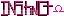-=+=- -=+=- -=+=- -=+=- -=+=- -=+=- -=+=- -=+=- -=+=- -=+=- -=+=- -=+=- -=+=- -=+=- -=+=- -=+=- -=+=- -=+=- -=+=- -=+=- -=+=- -=+=- -=+=- -=+=- -=+=- -=+=- -=+=- -=+=- -=+=- -=+=- (c) WidthPadding Industries 1987 0|493|0 -=+=- -=+=- -=+=- -=+=- -=+=- -=+=- -=+=- -=+=- -=+=- -=+=- -=+=- -=+=- -=+=- -=+=- -=+=- -=+=- -=+=- -=+=- -=+=- -=+=- -=+=- -=+=- -=+=- -=+=- -=+=- -=+=- -=+=- -=+=- -=+=- -=+=-
SoCoder -> Blogs Home -> Blogs

NolanCreated : 16 September 2009
Edited : 16 September 2009
System : Mac

### NolanDC.com

After a few months of having no time, I managed to get NolanDC.com up and working. Currently 'Articles' is the only fully-functioning part, but the others will follow soon. 'Projects' are working in development, but I'd like to make a few more tweaks.

The site and content management system were created from scratch in Ruby on Rails. I'm very happy with the result so far -- perhaps I'll post a picture of how simple the admin view looks.

Future possibilites:
• Development area, sort of a sandbox for web-dev
• Gallery of pictures
• Links page with handy tools
• Elements of design page, where I upload screenshots of website elements that appeal to me. This can be seen on smileycat.com

Any suggestions or ideas? Leave a comment (on the site) if you'd like. Also, I've only tested this in Firefox/Linux, and it'll remain that way until the release of Windows 7. That said, if you see any problems, let me know!

Wednesday, 16 September 2009, 14:37
SchererererHmm... gives me an error when I try to comment "ActionController::InvalidAuthenticityToken in CommentsController#create"

That was on Vista/IE8. It otherwise displays fine and everything in both IE8 & FF on Vista. Keep up the good work!Wednesday, 16 September 2009, 14:52
JayenkaiCool!

Keep up the work, and I'm sure it'll all be finished soon enough. Then you have to keep it all active! That's the harder partWednesday, 16 September 2009, 15:53
NolanThanks Jay, Scherer!

That seems like strange error. I can't seem to reproduce it, but I'll look into it further tonight.